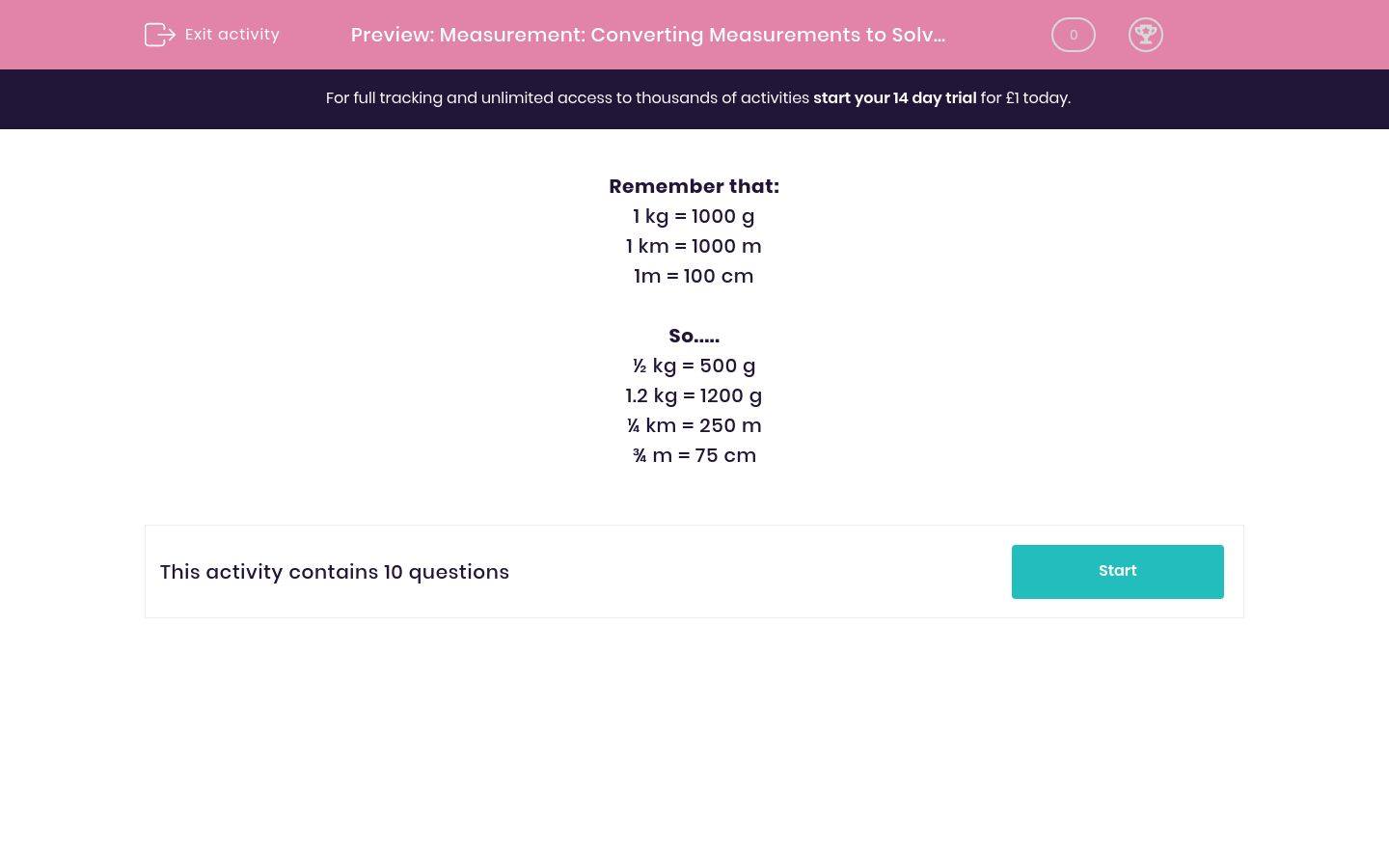# Measurement: Converting Measurements to Solve Problems

In this worksheet, students convert between metric measurements and answer measuring problems.Key stage:  KS 2

Curriculum topic:   Maths and Numerical Reasoning

Curriculum subtopic:   Metric & Imperial

Difficulty level:### QUESTION 1 of 10

Remember that:

1 kg = 1000 g

1 km = 1000 m

1m = 100 cm

So.....

½ kg = 500 g

1.2 kg = 1200 g

¼ km = 250 m

¾ m = 75 cm

A tree grows to a height of 5.5 metres.

Write this height in cm.

(just write the number)

Who is taller?

Jack is 1.2 m tall

Jill is 118 cm tall

Work out ½ kg + ¼ kg, giving your answer in grams.

(just write the number)

A 1 kg bag of flour is opened and Ben uses 450 g to bake a cake.

How much flour is left in the bag?

450 g

550 g

0.55 kg

0.055 kg

A cat has a mass of 3.5 kg

Write this mass in g.

(just write the number)

A cat has a mass of 3.5 kg and a dog has a mass of 5¼ kg

Write their total mass in kg.

(just write the number)

A sunflower is 2.4 m tall.

In one week, it grows 50 cm.

What is its height at the end of this week in metres.

(just write the number)

Work out 1 km - 50 m.

(just write the number)

A box of chocolates has a mass of 1.4 kg.

700 g is eaten after dinner.

Write down the mass of chocolates which remain in kg.

(just write the number)

I cycle 750 m and then walk 1.5 km.

How far have I travelled altogether in metres?

(just write the number)

• Question 1

A tree grows to a height of 5.5 metres.

Write this height in cm.

(just write the number)

550
• Question 2

Who is taller?

Jack is 1.2 m tall

Jill is 118 cm tall

Jack
• Question 3

Work out ½ kg + ¼ kg, giving your answer in grams.

(just write the number)

750
• Question 4

A 1 kg bag of flour is opened and Ben uses 450 g to bake a cake.

How much flour is left in the bag?

550 g
0.55 kg
• Question 5

A cat has a mass of 3.5 kg

Write this mass in g.

(just write the number)

3500
• Question 6

A cat has a mass of 3.5 kg and a dog has a mass of 5¼ kg

Write their total mass in kg.

(just write the number)

8.75
• Question 7

A sunflower is 2.4 m tall.

In one week, it grows 50 cm.

What is its height at the end of this week in metres.

(just write the number)

2.9
• Question 8

Work out 1 km - 50 m.

(just write the number)

0.95
• Question 9

A box of chocolates has a mass of 1.4 kg.

700 g is eaten after dinner.

Write down the mass of chocolates which remain in kg.

(just write the number)

0.7
• Question 10

I cycle 750 m and then walk 1.5 km.

How far have I travelled altogether in metres?

(just write the number)